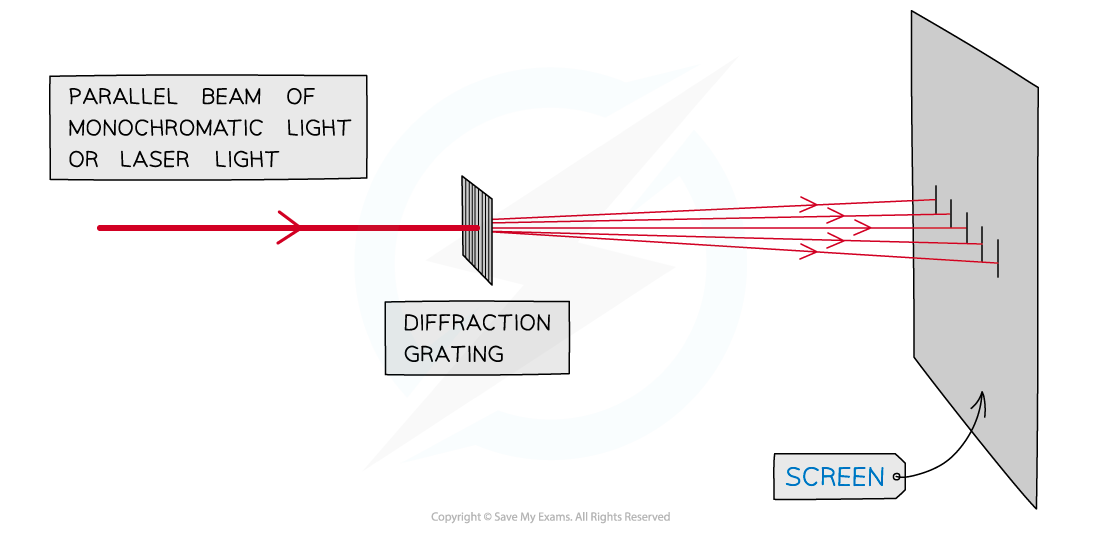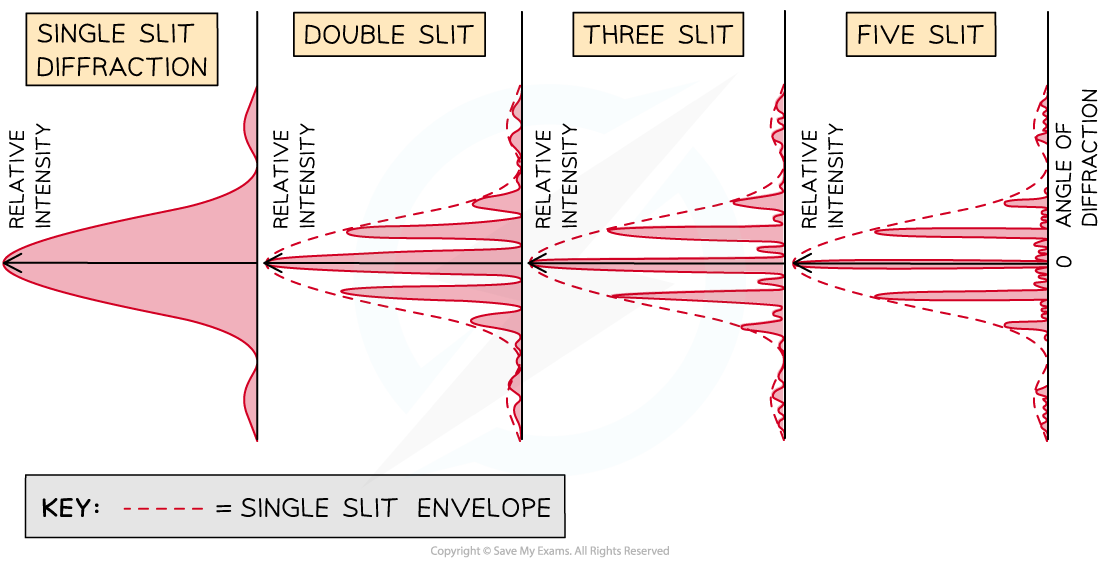# IB DP Physics: HL复习笔记9.3.3 Diffraction Grating Patterns

### Diffraction Grating Patterns

• A diffraction grating is a plate consisting of a very large number of parallel, identical, close-spaced slits
• When monochromatic light is incident on a grating, a pattern of narrow bright fringes is produced on a screenDiffraction grating with multiple slits, usually described in terms of 'slits per metre'

• The number of slits dictates both the interference and diffraction patterns which are seen
• On the screen a diffraction pattern is observed
• The interference pattern is a measure of the intensity of the light at different angles of diffraction away from the central maxima
• For a double slit arrangement this is shown in (9.3.2 Two-Slit Interference Patterns)
• On the diffraction pattern as the number of slits increases:
• Between the maxima, secondary maxima appear
• The central maxima and subsequent bright fringes become narrowerDiffraction patterns for light interfering through different numbers of slits

• When there are 3 slits, 1 secondary maxima can be seen between the primary maxima
• When there are 5 slits, 3 secondary maxima can be seen between the primary maxima
• Therefore, with N slits (when N > 2), there are (N − 2) secondary maxima
• Once the number of slits increases to N > 20:
• The primary maxima will become thinner and sharper (since slit width, [Image_Link]data:;base64,